# Battery charge time calculator

This tool calculates the maximum battery charge time for any given rechargable NiMH (Nickel-Metal Hydride) and NiCad (Nickel-Cadmium) battery.

How this tool works:

1. Get your rechargeable battery and find its battery capacity marked on the battery itself. It is the value ending with mAh (milliampere hours) or Ah (ampere hours). In the example below, the battery capacity is 1000 mAh.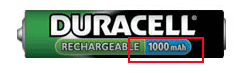An overview of different rechargeable battery sizes and their capacities.
These capacities may differ on your battery.

 Battery size AA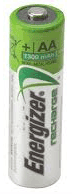1300mAh Battery size AAA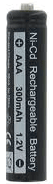300mAh Battery size 9V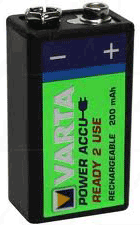200mAh Battery size C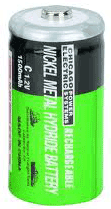7500mAh Battery size D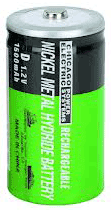7500mAh
Note: The battery images are not on scale.

2. Get your battery charger and find its charge current. It is the value ending with mA (milliampere) or A (ampere).

Example 1

Battery charger has 4 AAA battery bays.
On the charger there is a label with the following information: Min 90mA - Max 145mA
Which means:
Charging 1 AAA battery = 90 mA
Charging 2 AAA batteries = 90 mA + 1/3 * (145-90) = 108.33 mA
Charging 3 AAA batteries = 90 mA + 2/3 * (145-90) = 126.67 mA
Charging 4 AAA batteries = 90 mA + 3/3 * (145-90) = 145 mA

Example 2

Battery charger has 2 9V battery bays.
On the charger there is a label with the following information: Min 17mA - Max 42mA
Which means:
Charging 1 9V battery = 17 mA
Charging 2 9V batteries = 42 mA

3. The last step is to decide what the efficiency loss will be. The accepted standard for efficiency loss is 20%. Not all of the power applied to a rechargeable battery is put into the battery. Some of the power is lost e.g. as heat or battery inefficiency itself. This energy loss results in a longer charging time.

4. Now we have all information to calculate the estimated charge time:

AA battery capacity = 1000 mAh
Charge current = 90 mA
Efficiency loss = 20% (battery is recently bought and fully discharged)

Estimated charge time (excl efficiency loss) = Battery capacity / charge current
Estimated charge time (excl efficiency loss) = 1000 / 90 = 11.11 hours
Estimated charge time (incl efficiency loss) = Estimated charge time * (1 + (Efficiency loss / 100))
Estimated charge time (incl efficiency loss) = 11.11 * (1 + (20 / 100)) = 13.33 hours

Note:
The calculation is based on a fully discharged battery.
A battery that is only partially discharged needs to be recharged in less time.

## Battery charge time calculator input:

 Battery capacity *:mAh Ah Charge current*:mA A Efficiency loss *:20 30 40 50 % * = required

No output